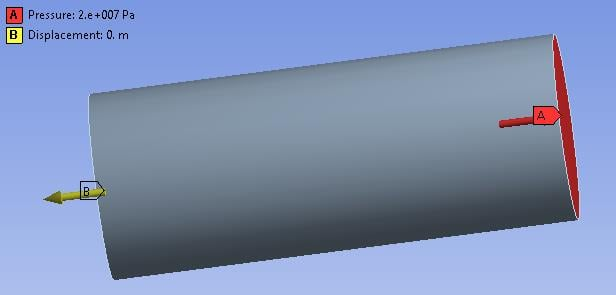## General Mechanical

•Niss
Subscriber

Hello,

I'm new to Ansys mechanical and I couldn't find a clear explanation on the internet about the types of support in Static structural.

I am confused about the different kinds of support: what is a fixed support versus a 0 displacement? What are the assumptions in each cases. Is it possible to have access to the equations underlying these constraints?

I tried to simulate a tensile test and see what difference it would give using a fixed support or a 0 displacement at the face normal to the load (on the other end) but Ansys does not run in the 0 displacement case...Also what would be the consequences of adding both symmetry and 0 displacement on the same face?

Thanks

•peteroznewman
Subscriber

All the descriptions below apply to solid elements, not shell elements.

Fixed Support means X, Y and Z are all set to 0.

If you have a plane of symmetry, that sets the displacement normal to that plane to 0.

If the normal to a plane of symmetry was the Z axis, and you put both symmetry and a Z=0 displacement constraint on the face, the result would be that the nodes on that face would have a Z=0 constraint. There is no problem specifying the same constraint twice.

If you have a cylinder and you slice it twice to make a quarter cylinder and have symmetry conditions on the two symmetry planes (say with an X and a Y normal), and you apply Z = 0 to the end face, you have fully constrained the quarter cylinder and can pull on the other end with a force or pressure.

This will allow the Z=0 end to slide in the X and Y directions as the Poisson's ratio takes effect and will result in a nice uniform stress at that end. This is a lot different to the full cylinder that has a fixed support. The face cannot move as the Poisson's ratio dictates and stresses are created because of that.

If you have a full cylinder and only use Z=0 instead of a fixed support, the solver cannot find equilibrium because X and Y are free and it doesn't know where to put them. This is resolved in the quarter model using two planes of symmetry.

•Niss
Subscriber

Thank you!

•tofuu88
Subscriber

Hi Peter

I intentionally created an account to tell you just how amazing all of your responses have been on this forum.   I genuinely appreciate it.    I don't know how to privately message someone on this forum so would you be willing to send me an email at ziyandennischen@gmail.com and let me know how to connect with you on Linkedin?

I just want to tell you how much I appreciate people like you.

Sincerely

Dennis